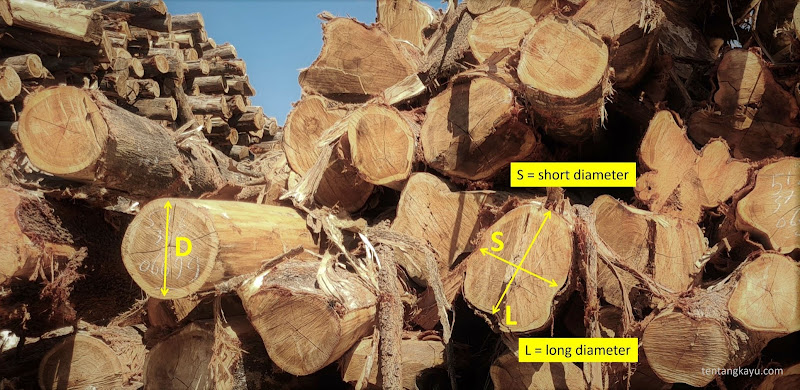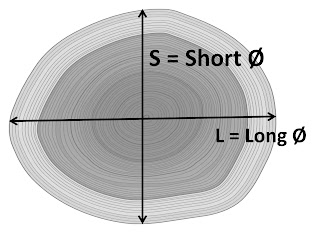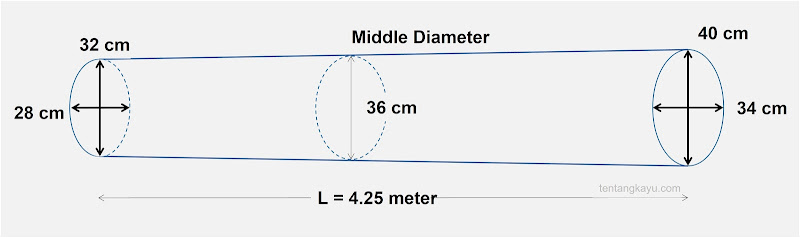# Estimate Log's Volume, Which One is the Most Accurate?Estimate the volume of logs oftenly there are polemic and debates to decide which one is right and the most accurate. The formula itself are quite simple and easy to do but on the site it's pretty complicated because the log shape is not always cylindrical. Even if you have perfect round shape, it has different diameter on the other end which can be smaller or larger.

Moreover if the cross-section shape of the log tends to be oval or ellipse, or even irregular like a star shape.
Basically, all formula available are correct. You just need to know which formula is more suitable for different log shape and wood species.

Referring to the cylinder like log shape, the main formula to measure the volume is:

V = π x r² x L
or
V = ¼ π x D² x L

Description:
V = Volume
π = Phi (22/7 or 3,14)
D = Diameter (cm)
L = Length (mtr)

Here are some calculation methods you can use.

Brereton
The Brereton formula focuses on the log diameter. The measurement of the average diameter (D) must be carried out 2 perpendicular measurements (S and L) under the bark at each log end. The measurement must record the average of both measurements in centimeter.
The length of the log shall be measured along a straight line parallel to the center axis of the log and shall be rounded to the nearest lower number.

V = 0,7854 x D² x L ÷ 10.000

Description:
D = Diameter (cm)
ØS = Short Diameter (cm)
ØL = Long Diameter (cm)

The number 0,7854 is from 3,14 (phi) divided by 4, as the conversion from radius measurement to diameter measurement.Smalian
The Smalian formula pays more attention to the cross-sectional area, by calculating the average surface area of the two log ends with log length. First, to calculate the cross-sectional area of the logs from both ends, then the results of the calculations are added up, and divided by 2 to get average surface area. Only then multiplied by the log length.

V = (area A + area B)/2 x L ÷ 10.000

Japanese Agriculture Standard (JAS)
The Japanese Ministry of Agriculture and Forestry has another way of calculating it. The log length is measured in meters (up to one tenth of a meter), and is rounded down. For example, if the log length is 235 centimeters, it will only be calculated as 2.3 meters.
The log diameter is measured only at the smallest diameter (S = short diameter). but if the difference between S and L diameter reaches 6 cm and multiples thereof, then 2 cm will be added to the short diameter measurement.

V = 0,7854 x ØS² x L ÷ 10.000

Huber
Huber's formula uses a slightly different approach to the Smalian formula which uses the log cross-sectional area for calculations. Huber's formula takes into account the size of the smallest diameter and the largest log diameter at the other end by adding up the two sizes and then dividing by 2. And then multiplied by the log length.
Huber can also use the average diameter at the center of the log.

V = (Ø short + Ø long)/2 x L ÷ 10.000

Estimation exercise
Let's compare some measurement results of the formulas above by using a log size illustration as the sketch below.Brereton Formula:
D average = (28 + 32 + 34 + 40cm)/4 = 33,5 cm
L = 4,2 meter
V = 0,7854 x (33,5 x 33,5) x 4,25 ÷ 10.000
V = 0,3746 m2

Smalian Formula:
Average Diameter A = (28 + 32) ÷ 2 = 30 cm
Average Diameter B = (40 + 34) ÷ 2 = 37 cm
Area A = 0,7854 x (30cm)² ÷ 10.000 = 0,0706 m2
Area B = 0,7854 x (37cm)² ÷ 10.000 = 0,1075 m2
Average area = (0,0706 + 0,1075) ÷ 2 = 0,08905 m2
Volume = 0,08905 x 4,25 m
V = 0,3784 m3

JAS Formula:
ØS = 28 + 4 cm (because there is +12 cm compared to ØL) = 32 cm
V = 0,7854 x (32 x 32) x 4,2 ÷ 10.000
V = 0,3377 m3

Huber Formula:
D = (28 + 40)/2 = 34 cm
V = 0,7854 x (34 x 34) x 4,25 ÷ 10.000
V = 0,3858 m3

If we sort the results above, the Huber formula produces the highest volume and JAS formula produce the lowest volume. We cannot conclude which formula is the most accurate or which one is wrong. All formulas are correct in their application based on basic calculations for the volume of a cylindrical shape.
That's why we must be able to use every different formula for the right type of application.

The calculation method from JAS shows the smallest results, but the estimation is more precise and workable for you to calculate raw material needs. With a small volume, indirectly it takes into account the waste percentage and unusable log & timber defects from production.
Or it can be more workable to calculate logs from softwood species, such as Albasia, because of the large % wood shrinkage.

The calculation results with the Huber formula are closer to the actual log volume, this is very appropriate if the log is used for the production of veneers or other artificial boards whose raw materials are almost 100% depends on the log shape.
Also it is very useful to estimate numbers of volume logs in the container where usually are smaller size for shipping using containers.

To our opinion, the Brereton formula is more practical and versatile for various purposes, whether in the log trading industry, preparation of production materials in factories, or for industries that provide sawmill services.